# SSAT Elementary Level Math : How to find the decimal equivalent of a fraction

## Example Questions

← Previous 1 3 4 5 6 7 8 9 18 19

### Example Question #1 : Fractions

What number is in the tenths place of 2.46?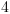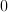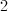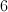Explanation:

The tenths place is the place to the right of the decimal point.

### Example Question #2 : Fractions

What number is in the tens place in 123.4?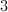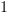Explanation:

The tens place is the second place to the left of the decimal.  The ones place is the place just to the left of the decimal.  The second number to the left of the decimal is 2.

### Example Question #1 : Fractions

Put the following numbers in order from least to greatest: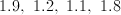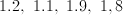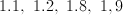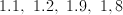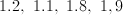Explanation:

All of these numbers are between 1 and 2.  Since they all have a 1 in the ones place, we just compare the tenths place digits and put them in order from least to greatest.

The tenths places here are 1, 2, 8, and 9.

So 1.1, 1.2, 1.8, 1.9.

### Example Question #2 : Fractions

Find the probability of the outcome. Express the outcome as a fraction, reduced to its lowest terms.

Sarah saw a basket full of apples. There were 17 green apples, 13 red apples, and 15 yellow apples. What is the probability that Sarah will choose a yellow apple if she picks one at random?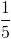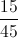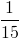Explanation:

To find the probability of an outcome, set up a fraction of the part and the total possible.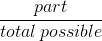There are 15 yellow apples, but we need to find the total possible by adding all the apples together.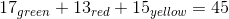Since there are 15 yellow apples (part) out of 45 apples in all (total possible) the fraction will be 15 over 45.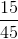We can reduce the fraction by dividing both parts by 15.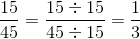### Example Question #1 : Fractions

Which fraction is less than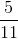?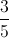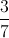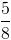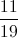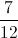Explanation:

Half of 11 is 5.5, so we know that this fraction is a little bit less than one half.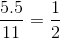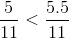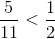We are looking for another fraction that is less than one half.

Half of 12 is 6, so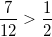.

Half of 8 is 4, so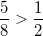.

Half of 5 is 2.5, so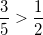.

Half of 19 is 9.5, so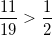.

Half of 7 is 3.5, so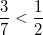.

The only answer that could possibly be less thanis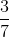.

### Example Question #6 : Fractions

Find the decimal equivalent to the fraction. Round to the nearest tenths place, if necessary.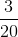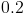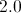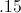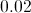Explanation:

When converting a fraction to a decimal, divide the denominator by the numerator. Divide 3 by 20. The quotient is 0.15. The question asked you to round to the nearest tenths place, so the correct choice is: 0.2.

### Example Question #7 : Fractions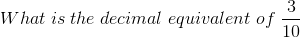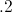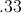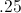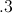Explanation: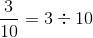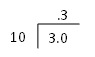### Example Question #8 : Fractions

What isequal to in decimal form?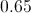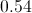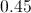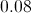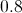Explanation:

To find the decimal equivalent of a fraction, divide the top number (numerator) by the bottom number (denominator). In this problem, 4 divided by 5 equals 0.8.

### Example Question #9 : Fractions

What is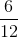equal to in decimal form?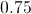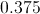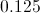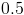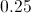Explanation:

To find the decimal equivalent of a fraction, divide the top number [numerator] by the bottom number [denominator]. In this problem, 6 divided by 12 equals 0.5.

### Example Question #10 : Fractionsstudents were asked to name their favorite superhero.answered Batman,answered Spiderman, andanswered Superman. What percentage of the students gave Superman as their answer?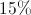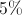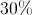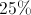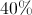students out ofanswered Superman.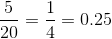, which is.